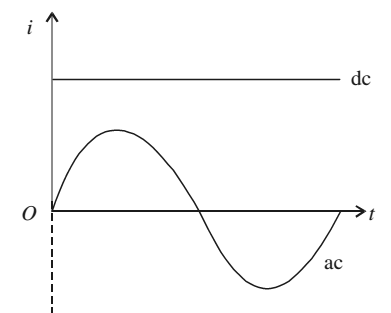# Alternating Currents and Voltages

When a battery is connected to a resistor, charge flows through the resistor in one direction only. If you want to reverse the direction of the current, you have to interchange the battery connections. However, the magnitude of the current will remain constant. Such a current is called direct current.

A current whose magnitude changes continuously and direction changes periodically, is said to be an alternating current.In general, alternating voltage and currents are mathematically expressed as

V = Vm cos ωt

I = Im cos ωt

Vm and Im are known as the peak values of the alternating voltage and current respectively.

The root mean square (rms) values of V and I are given as

Vrms = Vm/√2 = 0.707 Vm

Irms = Im/√2 = 0.707 Im

The relation between V and I depends on the circuit elements present in the circuit.